• ### Assistance with select function needed...

Hi all,
I'm struggling to make something work, any suggestions would be much appreciated. I'm very new to PD so if I'm doing anything wrong that's blindingly obvious please let me know! Oh and sorry if this is posted in the wrong place.

I'm making a module to trigger a drum machine using bangs using an osc~, i.e selecting different points along the 1 to -1 wave to trigger from. I thought I'd use osc~ as I need to easily reset to the start of my pattern, given osc~ has a phase reset this made sense in my head.

For some reason, the select box is not selecting a value (I chose 0.2 at random) from the snapshotted and rounded (to 2 d.p) osc~ output. It is however behaving normally if I send a message to it of 0.2.

Any suggestions?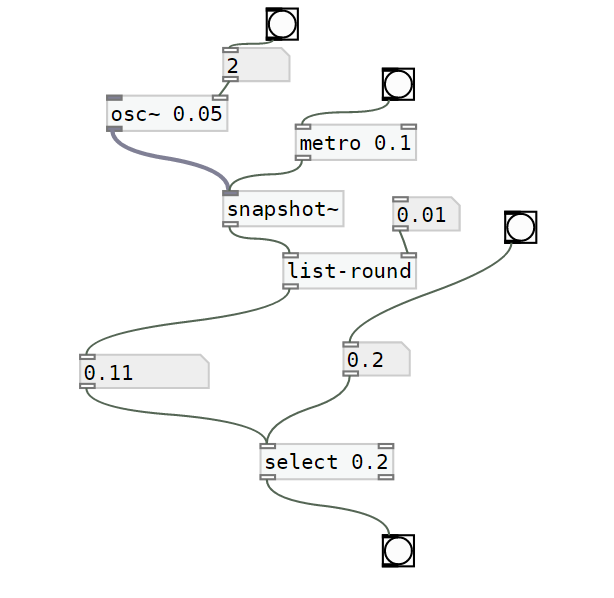Many thanks

• | Posts 5 | Views 341
• @Ikeobob I don't know why an "is it equal" test fails with fast changing floats even when the value is printed numerous times.
Someone clever will tell us...... @jancsika
A "greater than" or "less than" test will work, and the [change] object will force the test to trigger only once....... but only on the rising part of the [osc~] curve with a "greater or equal" test.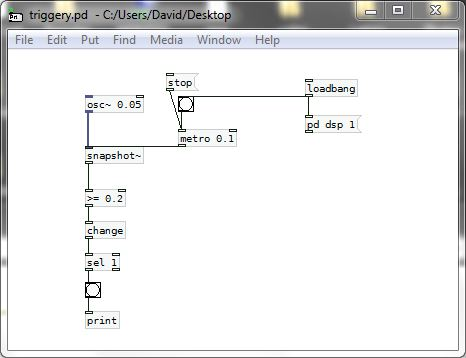SO........ that gets complicated as for a trigger on other parts of the curve a different test would be needed.
The other problem you will have with [osc~] for this application is that a sine wave is not giving you a linear output.... so for timing purposes you are lost.....You should use a sawtooth wave instead........ so [phasor~] is a better friend than [osc~]...... and as there are only positive values the test can stay the same. You are simply choosing a point on the ramp, and it is linear......
triggery.pd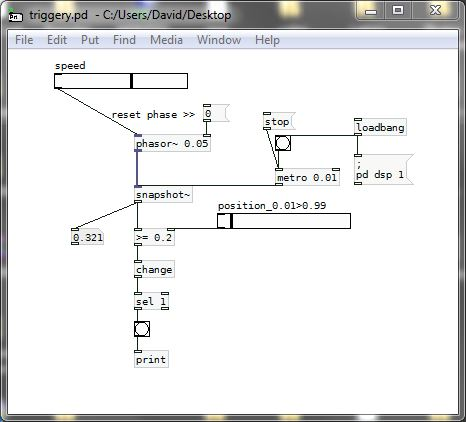And then you could be better off using [line] to trigger as the cpu use should be less.

triggery2.pd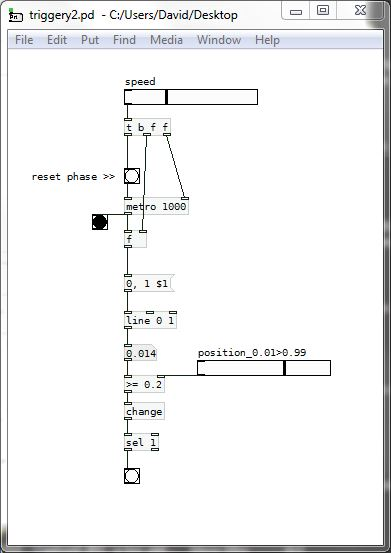AND...... much better.......If we scale up the "equals" test to integers then we can test from zero to one and [select] works.......
It should also work with the [phasor~] example above...... BUT..... with [phasor~] you would have to scale in a way that makes sure that a match occurs and that you do not get more than one trigger per cycle.
triggery3.pd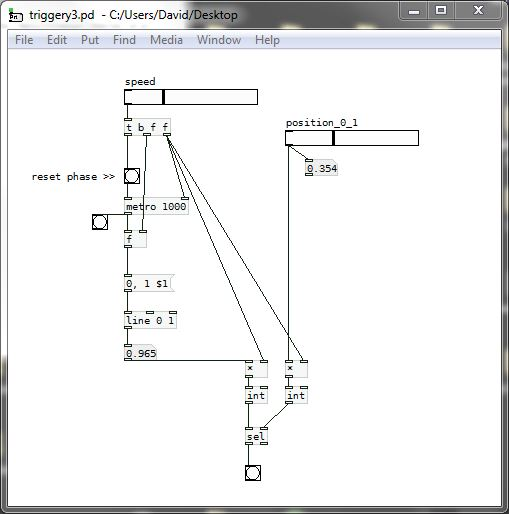David.

• You've entered the fun and exciting world of floating point fractions.

0.2 is a finite decimal, but in binary, it's infinitely repeating. (1/3 in base 10 is infinitely repeating, because the denominator includes at least one prime factor, 3, which is not a prime factor of the base. Decimal fractions are finite only if the denominator has no prime factors other than 2 or 5: 5, 10, 20, 2, 4, 8 etc. are OK, 3, 7, 11, 15 are not.)

In binary, the base has only one prime factor: 2. So a fraction is exact only if the denominator is a power of two. 0.2 = 1/5 = non-power-of-two denominator.

So when you write 0.2, the floating-point number is something very close to 1/5 but not exactly. And the rounding algorithm is coming up with some other floating point number that is very close to 1/5 (but again, not exactly, because 0.2 is impossible to represent exactly in binary).

TL;DR "equals" comparisons for floating-point numbers are not necessarily safe.

You could multiply the osc~ by 100, round to 1.0, and [select 20]. Then the denominator is 1, which is a (trivial) prime factor of base 2, therefore non-repeating, therefore exact.

hjh

• @whale-av many thanks!! This makes sense• @ddw_music likewise, thank you! Fascinating stuff

| Posts 5 | Views 341
Internal error.

Oops! Looks like something went wrong!